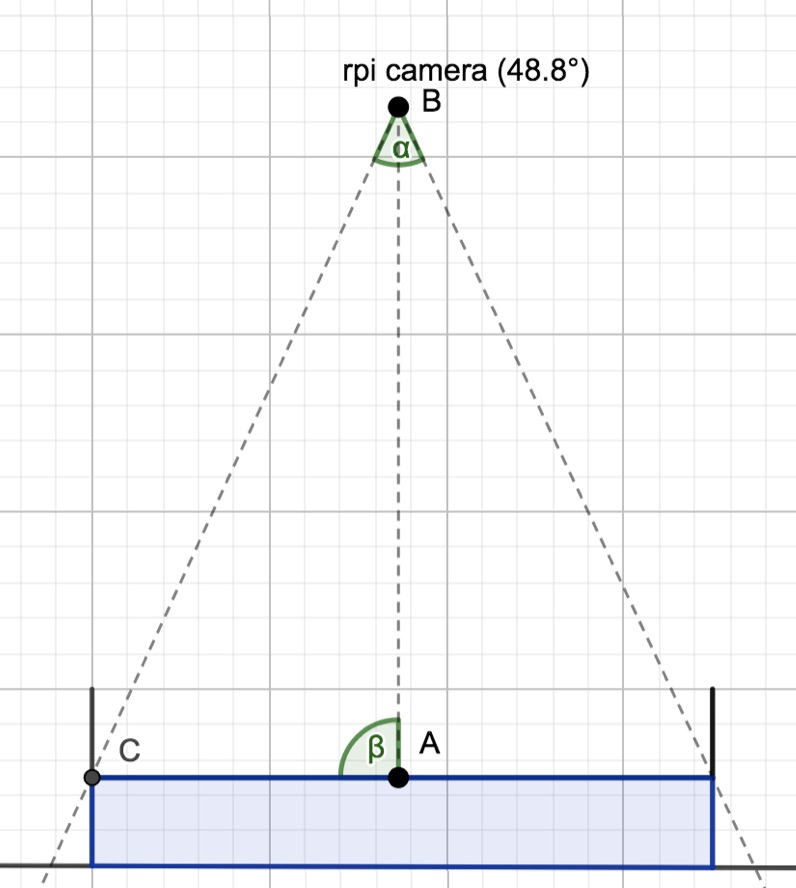# Optimal positioning of the Raspberry Pi camera

In this guide I will help you optimally position the Raspberry Pi Camera

## Overview

For optimal positioning of your Raspberry Pi camera, it is often best to place the camera as close as possible to the area you want to record just until only the area is in the camera’s field of view. Although this seems quite complicated at first, with a couple parameters and some simple formulas, we can easily calculate the optimal position of the camera.## What we need

First of all, we will need the dimensions of the area you are recording and the camera angle. The v2 Camera has a horizontal field of view (fov) of 62.2 degrees and a vertical fov of 48.8 degrees (link). You should try and orient the camera in such a way that the longest part of the area you are recording is the horizontal camera axis.

## Calculations

We can simply use Pythagoras to determine the distance from the camera (AB) if we know the camera angle (alpha) and the plane in view (half the arena). In R we can create a function to calculate the distance as follows:

``````calcdist <- function(angle_of_view, plane_of_view){
result <- (plane_of_view / tan((angle_of_view * pi / 180) / 2)) / 2
result <- round(result,3)
cat("Distance to plane =", result, "\n")
}
``````

## An example

Now we can calculate the minimum camera distance to have the full area in its field of view. Let’s say we want to record an aquarium to film the behaviour of fish from above. The aquarium will be 70cm by 40cm and have a water depth of 10cm.

We want to use the horizontal camera axis to film the long side of the tank and the vertical axis to film the short side, so adding the values to the function:

``````calcdist(62.2, 70)
calcdist(48.8, 40)
``````

will show as output:

``````Distance to plane = 58.02
Distance to plane = 44.09
``````

So the camera should be at 58+10 = ~ 70cm above the tank bottom. Note that in this case the camera should be positioned exactly about the tank centre and directly pointing down, as otherwise part of the tank may not be properly in the field of view.